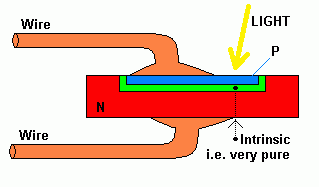RANDOM PAGE

SITE SEARCH

LOG
IN

HELP

# PIN Diodes

This is the AQA version closing after June 2019. Visit the the version for Eduqas instead.

## Construction

PIN Diodes are used as photo detectors and for high frequency radio switches and attenuators.

The diode has three layers.

• A very thin P type layer - so thin, photons get through.
• Very pure or "Intrinsic" semiconductor.
• An N type layer.
• hence PIN.## How it Works

When the device is reverse biased, there is a minute leakage current. If light (photons) hit the Intrinsic Layer, electrons are dislodged and this leaves a hole. The electrons migrate towards the Anode (positive) and the holes migrate towards the Cathode (negative). So light shining on the device increases the leakage current.

If the PIN diode is reverse biased with a very high voltage, when an electron is dislodged, it accelerates towards the anode and this dislodges more electrons which also accelerate. This causes an avalanche effect and quite a large current pulse flows even if only one photon triggered the avalanche.

Ten gigabits per second data transfer rates are possible because these diodes respond so fast.

## Calculation Example

Diode Characteristics ...

 Dark leakage current 5nA Sensitivity 1.2 A/W 1.2 amps per Watt. So 1 microwatt of light would produce a leakage of 1.2 microamps. Capacitance at zero bias 8pF Reverse biased diodes behave like capacitors. Between the P and N layers there is s depletion zone where there are no charge carriers. So there are two conducting zones separated by an insulating zone. This structure is lust like a capacitor. It gets more interesting. As the reverse bias is increased, the depletion zone gets bigger so the capacitance decreases. It's like increasing the plate separation of a capacitor. Response time to 95% output 5nS As the diode also behaves like a capacitor, this circuit behaves like an RC timing circuit. This limits the speed at which the diode can switch. A smaller capacitance increases the switching speed. A higher reverse bias voltage reduces the capacitance. The breakdown voltage must not be exceeded.### Dark Output Voltage

Use the dark leakage current for this calculation.

V    =    I R    =    5x10-9 x 2.2x106    =    11mV

### Vout at 2µWatts Incident Light

The diode produces 1.2 Amps per Watt or 1.2 microamps per microwatt to the current will be just over 2 µA.

I    =    5x10-9 + 2x10-6 x 1.2    =    2.405µA

V    =    I R    =    2.405x10-6 x 2.2x106    =    5.29 V

### RC Time Constant at Zero Bias

T    =    R C    =    2.2x106 x 8x10-12    =    17.6 µs

The PIN diode responds much faster than this because it is not used at zero bias. The highest possible reverse bias is used. This greatly reduces the capacitance of the device.

### Circuit Sensitivity

To increase the sensitivity ...

• The resistor could be increased but this means the leakage current might no longer be negligible.
• Several diodes could be used in parallel to increase the current at a given light level.

reviseOmatic V3     Contacts, ©, Cookies, Data Protection and Disclaimers Hosted at linode.com, London